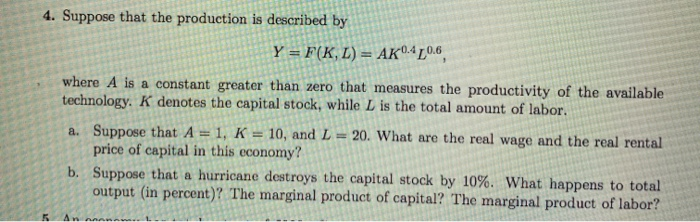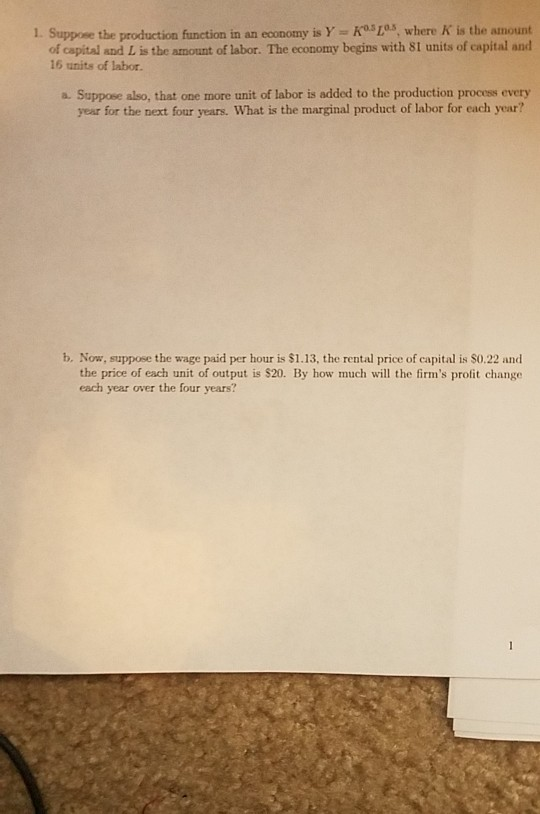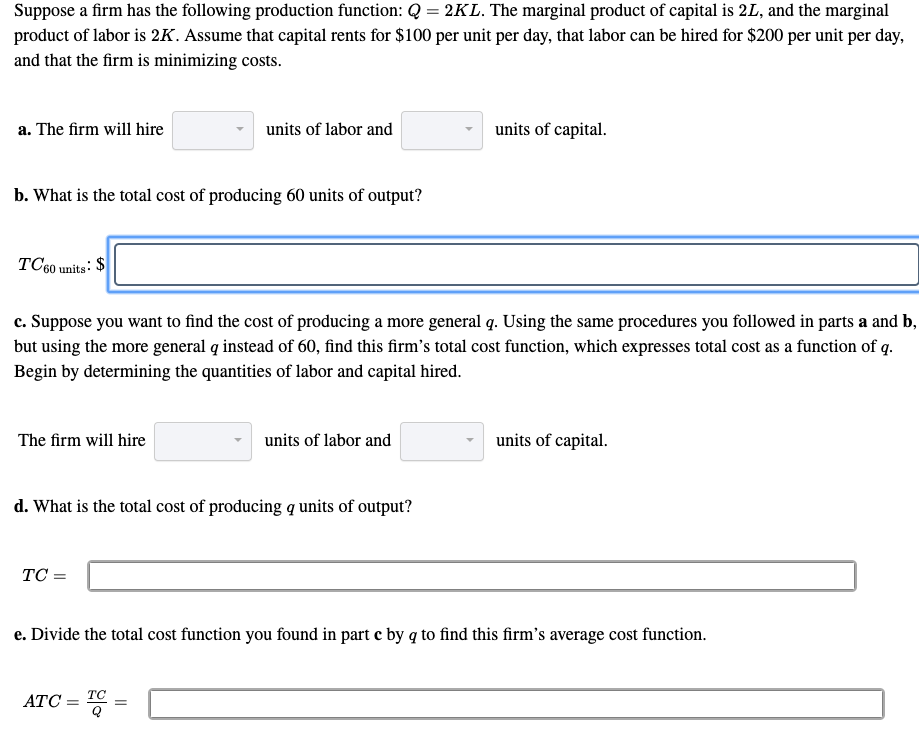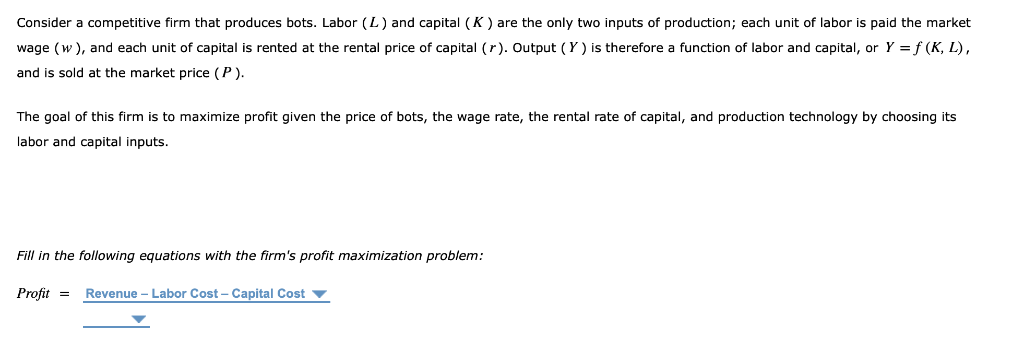Question

# Suppose a firm’s production function is Y=f(K,L) and has the following: Output = 5,000 Wage rate...

Suppose a firm’s production function is Y=f(K,L) and has the following:

Output = 5,000

Wage rate = 40

Marginal product of labor = 5

Labor = 100

Rental rate = 250

Capital = 75

Marginal Product of capital = 20

Price = 10

A. What is the firm’s total revenue?

B. What is the firm’s total cost?

C. What is the profit for the firm?

D. What is the real wage rate for this firm?

E. What is the real rental rate?

F. Suppose the firm is deciding whether or not to expand its factors of production. Do you recommend they hire another worker and/or add another unit of capital? What justification can you show to justify your answers? (Hint: I am looking for a yes or no for each factor and a mathematical justification for why you came to that conclusion)

A) Total revenue = Output * Price = 5,000 * 10 = 50,000

B) Total Cost = Labor Cost + Capital Cost = Wage rate * labor + rental Rate * Capital

= 40*100 + 250*75 = 22,500

C) Total Profit = Total revenue - Total cost = 50,000 - 22,500 = 27250

D) Real Wage Rate = Wage Rate/ Price = 40/10 = 4

E) Real rental rate = Rental rate/Price = 250/10 = 25

F) Ratio of Marginal Product of labor (MPL) to Marginal Product of capital (MPK) = 5/20 = 0.25

Ratio of Capital employed to Labor employed = 75/100 = 0.75

Therefore MPL/MPK < Capital Labor ratio. Therefore firm should hire more capital as capital is underemployed in relation to the employed.

So Hire Capital: Yes

Hire Labor = NO

#### Earn Coins

Coins can be redeemed for fabulous gifts.

Similar Homework Help Questions
• ### Suppose that a firm has a production function given by: ? = ?^?.???^?.? . The wage...

Suppose that a firm has a production function given by: ? = ?^?.???^?.? . The wage rate is \$18 and the rental rate is \$9. 12. Suppose that the firm has 4 units of capital in the short run. Find the short run total cost function. ________________________________ 13. Find the marginal product of labor (MPL) function. ________________________________ 14. Solve the optimization condition for K and write that equation. ________________________________ 15. Suppose the firm wants to minimize the cost of producing...

• ### 4. Suppose that the production is described by Y = F(KL) = AK0.40.6 where A is...4. Suppose that the production is described by Y = F(KL) = AK0.40.6 where A is a constant greater than zero that measures the productivity of the available technology. K denotes the capital stock, while L is the total amount of labor. a. Suppose that A = 1, K = 10, and L = 20. What are the real wage and the real rental price of capital in this economy? b. Suppose that a hurricane destroys the capital stock by...

• ### Suppose that a firm had a production function given by: q=L^0.25*K^0.75. The wage rate (w) is...

Suppose that a firm had a production function given by: q=L^0.25*K^0.75. The wage rate (w) is \$10 and the rental rate (r) is \$20. Calculate the amount of labor the firm would hire when it produces 300 units of output in a cost-minimizing way

• ### 3. Suppose a firm has the production function Q = 50 KL 1) If the wage...

3. Suppose a firm has the production function Q = 50 KL 1) If the wage rate is \$10 per unit of labor and the rental rate of capital is \$5 per unit of capital, how much capital and labor should the firm employ in the long run to minimize the cost of producing 40,000 units? 2) Using the solution in part 1), what will the firm’s long-run total cost be?

• ### 1. Suppose the production function in an economy is Y- of capital and L is the...1. Suppose the production function in an economy is Y- of capital and L is the amount of labor. The economy begins with 8I units of capital and 16 units of labor. a. Suppose also, that one more unit of labor is added to the production process every year for the next four years. What is the marginal product of labor for each year? b. Now, suppose the wage paid per hour is \$1.13, the rental price of capital is...

• ### Suppose a firm has a production function given by Q=2K+L, where L is labor, K is...

Suppose a firm has a production function given by Q=2K+L, where L is labor, K is capital and Q is the quantity of output. Which of the following statements is WRONG? A. The firm is exhibiting constant returns to scale B. The firm’s marginal product of capital is constant C. The firm’s marginal product of labor is constant D. The firm’s marginal rate of technical substitution depends on the amount of inputs

• ### Suppose a firm has the following production function: Q = 2K L. The marginal product of...Suppose a firm has the following production function: Q = 2K L. The marginal product of capital is 2L, and the marginal product of labor is 2K. Assume that capital rents for \$100 per unit per day, that labor can be hired for \$200 per unit per day, and that the firm is minimizing costs. a. The firm will hire units of labor and - units of capital. b. What is the total cost of producing 60 units of output?...

• ### Assume labor and machines are used in the production of an output.   The marginal product of...

Assume labor and machines are used in the production of an output.   The marginal product of the last worker hired was 175 units a day and the marginal product of the last machine employed was 850 units a day. The wage to hire a worker for a day is \$100 while the cost to employ the machine for a day was \$400. Assuming perfect competition what would you recommend the firm do to become more efficient?

• ### Consider a competitive firm that produces bots. Labor (L) and capital (K) are the only two...Consider a competitive firm that produces bots. Labor (L) and capital (K) are the only two inputs of production; each unit of labor is paid the market wage (w), and each unit of capital is rented at the rental price of capital (r). Output (Y) is therefore a function of labor and capital, or Y = f (K, L), and is sold at the market price (P). The goal of this firm is to maximize profit given the price of...

• ### Assume labor and machines are used in the production of an output.   The marginal product of the last worker h...

Assume labor and machines are used in the production of an output.   The marginal product of the last worker hired was 175 units a day and the marginal product of the last machine employed was 850 units a day. The wage to hire a worker for a day is \$100 while the cost to employ the machine for a day was \$400. Assuming perfect competition what would you recommend the firm do to become more efficient?